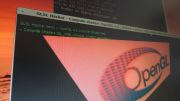Home Utilities Tutorials 3D Demos Graphics Cards Forums About

 GeeXLab
Current version: 0.29.17
>GeeXLab homepage

 FurMark
Current version: 1.21.1
>FurMark homepage

 GPU Caps Viewer
Current version: 1.44.2.1
>GPU Caps Viewer homepage

 GPU Shark
Current version: 0.16.1.0
>GPU Shark homepage

 Blogs
>JeGX's HackLab

 Geeks3D's Articles
>GPU Memory Speed Demystified

>How to Get your Multi-core CPU Busy at 100%

>How To Make a VGA Dummy Plug

>Night Vision Post Processing Filter

 PhysX FluidMark
Current version: 1.5.4
>FluidMark homepage

 TessMark
Current version: 0.3.0
>TessMark homepage

Current version: 0.3.0

 Demoniak3D
Current Version: 1.23.0
>Demoniak3D
>Libraries and Plugins
>Demos
>Codes Samples

3D Graphics Search Engine:Ambient Occlusion Lighting

By Jérôme 'JeGX' GUINOT
jegx [at] ozone3d [dot] net

Initial draft: November 19, 2005
Last update: February 9, 2006

Translated from french by Samir Fitouri - soundcheck [at] ozone3d [dot] net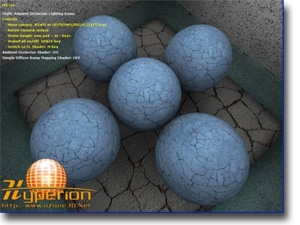1 - Introduction2 - The occlusion-map3 - AOGen - Occlusion-map generator4 - The Ambient Occlusion GLSL shader5 - Downloads

Prerequisite

1 - IntroductionAmbient Occlusion Lighting is a lighting technique which makes it possible to increase in a very appreciable manner the realism of a 3D scene. It can be considered as a lower cost solution to imitate the total illumination techniques that are usually used in ray tracing softwares.

To arouse your interest, here is an example of a 3d real time rendering result that exploits the Ambient Occlusion, resulting from the Static Ambient Occlusion Lighting Demo (called thereafter Static AO demo):Fig.1 - Ambient Occlusion rendering.

The AO technique (AO for Ambient Occlusion) is mainly focused on the ambient term of the lighting equation used in real time calculations. Here is the equation:
`If = Ia + Id + Is`

where If is the intensity of the pixel final color, Ia the intensity of the ambient color, Id the intensity of the diffuse color and Is that of the specular color. For more details regarding the calculation of the Id and Is terms, please refer to the Bump Mapping . tutorial.

In this equation, the Ia term is constant for a given 3d object. That means that if the lighting equation is reduced to If = Ia, the 3d object will be colored in a uniform color and we won't be able to distinguish its 3d morphology. Figure 2 shows the scene of the Static AO demonstration illuminated by a constant ambient intensity: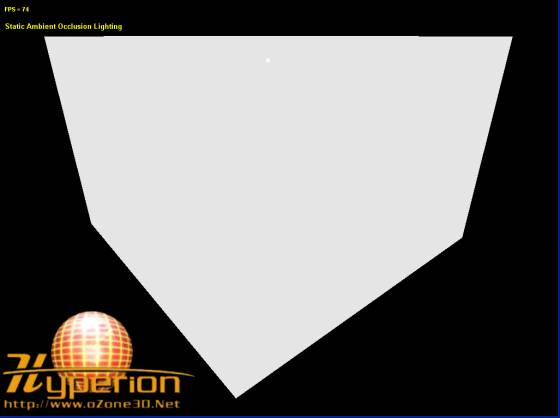Fig.2 - Constant ambient intensity rendering.

We can't see a thing. By correctly modulating this ambient intensity (with the occlusion factor which we will explain further) we obtain the result of figure 3: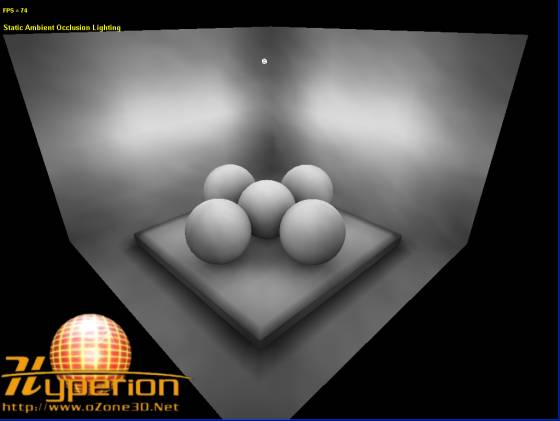Fig.3 - Modulated ambient intensity rendering.

And now the 3d objects of the scene appear very clearly. Therefore, an illustrated explanation of the AO would say that it consists in applying a variable modulation factor to the ambient intensity factor of the scene. That can be summarized by the following equation:
`Ia = IA x occ(v)`

where Ia is the final ambient intensity, IA the constant ambient intensity and occ() a function that depends on the vertex which gives the modulation factor.

Let us now start giving a soul to the occ() function.

2 - The occlusion-mapDescribing the occ() function with a strictly analytical method would be impossible (except perhaps for some very simple cases (???)). The solution to the occ() function problem is very simple: we just need to store the occlusion data for each vertex in a file named occlusion-map.

Once this file is created, we have to load in each vertex the corresponding data which we can then easily get back in the AO vertex shader. Well, dealing with vertex / pixel shaders is inevitable to implement sophisticated lighting techniques. But don't worry, the AO shader that we will describe will be easily reusable in your Demoniak3D demonstrations (or others)!

Let us see now the principle of occlusion-map creation as shown on figure 4:Fig.4 - Calculation of the occlusion term.

The calculation of the occlusion data is rather simple: starting from the center of a polygon, rays are projected in all directions in the higher hemisphere of the polygon. The occlusion factor (or term) which is the modulation factor previously considered, is defined as the ratio between the number of rays that did not touch any other polygon and the total number of launched rays. For example, if among 100 launched rays, 70 do not have any intersection with the other polygons of the 3d object, then the occlusion factor is equal to 70/100 = 0.7. The occlusion factor is a standardized value which lies between 0 and 1.

This process must be repeated for all the polygonal faces of the 3d model. Once the occlusion term for each face has been achieved, a fast calculation (compared to launched rays) enables us to get the occlusion term for each vertex of the 3D model. It is this value that will be stored in the occlusion-map.

Actually, significant information is stored in the occlusion-map: the bent-vector. This vector is just the average vector of all the launched radius vectors which did not intersect any face. Graphically, we can visualize this vector as being the central vector of the Non Occluded Area zone. This new average vector will replace the normal vector that is defined for each vertex in the lighting calculations.

The main point of the Ambient Occlusion Lighting realism lies in the combined action of the occlusion factor and the bent-vector.

Update: February 9, 2006:
The algorithm in pseudo-code for the ambient occlusion calculation is given in this topic in the oZone3D.Net benchmarking forum.

3 - AOGen - Occlusion-map generatorTo make things easier, I developed a little tool named AOGen that aims only to create an occlusion-map starting from a 3D model. The generated occlusion-map is a XML file and contains the bent-vector and the occlusion factor for each vertex of each 3d model's mesh. This XML file is absolutely not related to Demoniak3D and can be used in any 3d application that can read XML files and occlusion data as well.

For the moment, AOGen works as a command line tool, but a new version featuring a graphic interface may quickly be developed if needed by the users.

The creation of an occlusion-map with AOGen is done with the following syntax:
```C:\>AOGen <model_file> <occlusion_map_file> <num_samples>

model_file is the 3d model to be preprocessed. AOGen makes it possible to load the same 3d models as Demoniak3D, which are the * 3DS, * ASE, * OBJ, * FBX and * O3MDL file formats.

occlusion_map_file is the name to be given to the occlusion-map XML file.

num_samples is the number of rays to launch for each polygon. The greater is this number, the more accurate the occlusion data will be, and the longer the computing time will be! 128 samples seems to be a minimal value to get graphically acceptable results.

max_collision_dist makes it possible to exclude the polygons that are located too far away from the face being processed. max_collision_dist is the maximum distance for a polygon to be taken into account in the intersection test. A value of 100.0 for max_collision_dist gives quite good results (if 3D models are not too small!). In fact, num_samples and max_collision_dist are dependent on the 3d model and must be empirically adjusted.

num_threads makes it possible to launch calculation on one or more threads.

AOGen is multithreaded and thus makes it possible to exploit at best the multi-cores architectures of the new processors. The following table shows the computing times in seconds for processing the model of the Static AO demonstration, for 1 and 2 threads and this for two different workstations, the first equipped with a 3.6GHz P4 HT processor and the second with a 2.2GHz AMD X2 processor.

 Samples 1 thread 2 threads Profit 64 784 570 31% 128 1457 1201 17%

AMD Athlon X2 2.2GHz
 Samples 1 thread 2 threads Profit 64 620 332 46% 128 1212 671 45%

These tables tell us that AOGen fully expresses its potential on a true multi-core architecture like the AMD X2 processor. Indeed, in this case, the speed gain is about 50% between 1 thread and 2 threads. With an architecture based on the HT (HyperThreading) technology like that of the Pentium 4, the increase is about 25%.

The main problem of the current version of the AOGen utility is that it is too slow. Indeed it uses the bruteforcing for occlusion data calculation. Therefore, when dealing with high polygon 3D models, the computing times can become huge. I will try to implement as soon as possible a more advanced algorithm (based on the octrees or kd-trees) which would dramatically decrease the preprocessing time.

The following images show the importance of the number of rays (or samples numbers) for the accuracy of the occlusion data: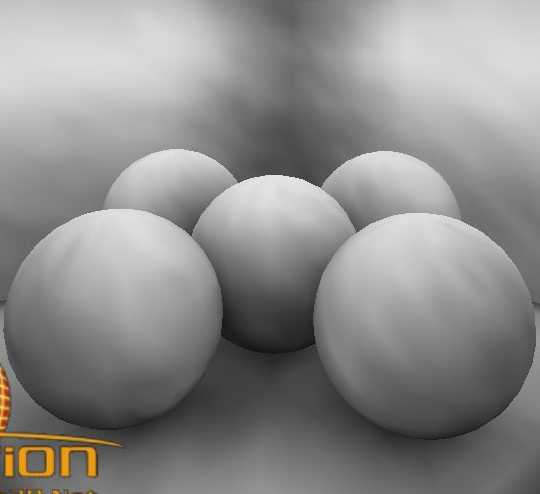Fig.5 - 64 samples per face.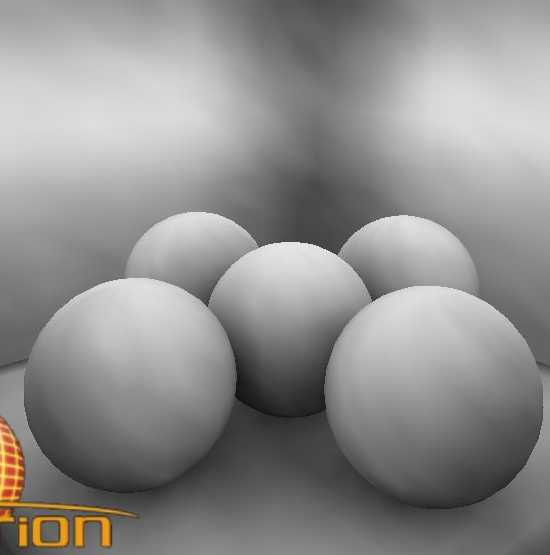Fig.6 - 128 samples per face.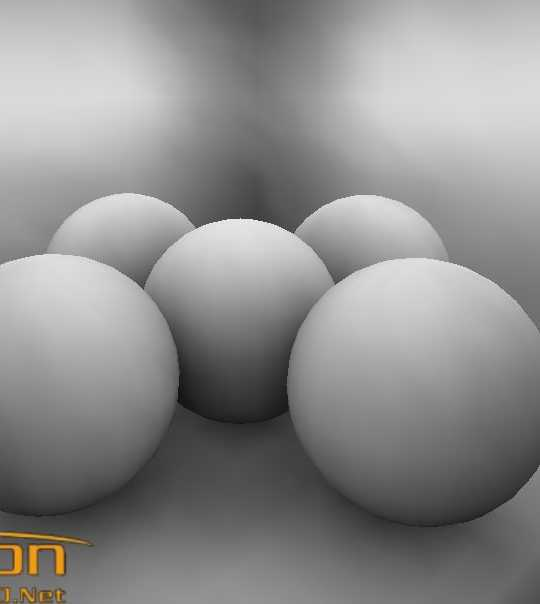Fig.7 - 256 samples per face.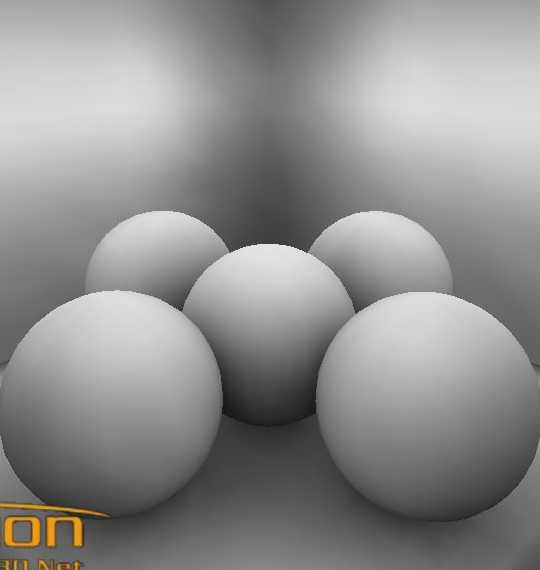Fig.8 - 512 samples per face.Fig.9 - 1024 samples per face. It is almost perfect!

Thanks to Satyr for putting his AMD X2 workstation at my disposal for preprocessing the scene into 512 and 1024 samples. Th4nX!

4 - The Ambient Occlusion GLSL shaderThe scene of figure 3 has been rendered with the GLSL (OpenGL Shading Language) shader according to:
```[Vertex_Shader]

attribute vec4 ambient_occlusion;
varying float ambient_occlusion_term;

void main(void)
{
gl_Position = ftransform();
ambient_occlusion_term = ambient_occlusion.w;
}

varying float ambient_occlusion_term;

void main (void)
{
vec4 vAmbient = vec4(0.9, 0.9, 0.9, 1.0);
vAmbient.rgb *= ambient_occlusion_term;
gl_FragColor = vAmbient;
}```

The occlusion data are stored at the level of each vertex as a vertex attribute. These vertex attributes are accessible in GLSLthrough the attribute type variables. For each vertex, we thus get the occlusion data in the ambient_occlusion variable. Theses occlusion data are a 4d vector. The x, y and z coordinates of the ambient_occlusion vector store the bent-vector, and the W coordinate stores the occlusion factor. This occlusion factor is then used in pixel shader to modulate the ambient intensity. In this shader, the bent-vector is not used.

This very simple shader does not have much utility except for this tutorial. For a real shader, I recommend you to have a look to the one of the Static AO demonstration which implements Ambient Occlusion Lighting with textures and bump mapping.

In Demoniak3D, it is important to clearly specify in which vertex attribute it is necessary to load the occlusion data. In fact, in case of bump mapping for example, the vertex attribute 1 already stores the tangent vector. In that case, it will be necessary to load the occlusion data in the next attribute, which is the attribute 2. The following piece of code shows the occlusion map loading in the model of the Static AO demonstration. The scene is rendered in bump mapping:
```<model 	name="temple" filename="data/scene_05.3DS" lighting="TRUE"
texturing="TRUE" use_vbo="TRUE" remove_seam="TRUE" >

<position x="0.0" y="0.0" z="0.0" />

<ambient_occlusion_lighting active="TRUE"
ambient_occlusion_map="data/scene_05_128_samples.xml"
vertex_attrib="2" />

compute_tangent_space_vectors="TRUE" auto_gen_mipmaps="TRUE" />

</model> ```

The XML vertex_attrib attribute of the ambient_occlusion_lighting element enables us to specify in which vertex attribute to load the occlusion data.

The technique presented in this tutorial is described as static (Static Ambient Occlusion Lighting) because the occlusion data for a model are once and for all calculated in an offline process. The 3d model should not undergo any transformations or deformations, otherwise the occlusion data will lose their significance. This type of ambient occlusion is perfectly adapted for the static 3d models such as houses indoors, constructions or arts (statues for example).[:: Static AO Demo ::]*** This demo requires Demoniak3D to be executed. Demoniak3D is available here: [:: Demoniak3D Demo-System ::].GeeXLab demosGLSL - Mesh exploderPhysX 3 cloth demo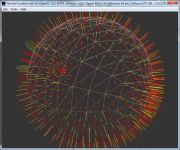Normal visualizer with GS